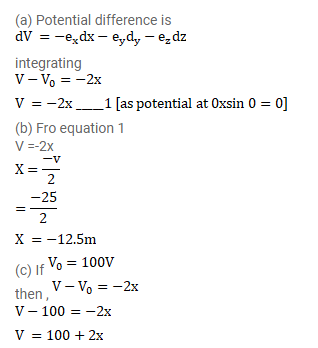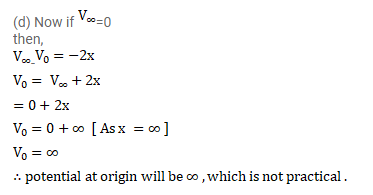# A uniform field of 2.0N/C exists in space in x-direction.`
Question:

A uniform field of $2.0 \mathrm{~N} / \mathrm{C}$ exists in space in x-direction.

(a) Taking the potential at the origin to be zero, write an expression for the potential at a general point ( $x, y, z$ ).

(b) At which points, the potential is $25 \mathrm{~V}$ ?

(c) If the potential at the origin is taken to be $100 \mathrm{~V}$, what will be the expression for the potential at a general point?

(d) What will be the potential at the origin if the potential at infinity is taken to be zero? Is it practical to choose the potential at infinity to be zero?

Solution: